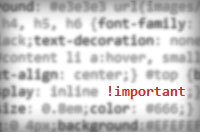In CSS, the “!important” suffix was originally intended to provide a method of overriding author stylesheets. Users could define their own “user stylesheets” and could use this suffix to give their rules precedence over the author’s (website creator’s) styles.

Unfortunately, and quite predictably, its usage has spread massively, but not in the right direction. Nowadays, it’s used to counteract the pain of having to deal with CSS specificity, otherwise known as the set of rules which dictate that “div h1 a” is more specific selector than “div a”. Most people that use CSS on a daily basis don’t know enough about CSS specificity to solve their problems without using the “!important” suffix.

For reference, “specificity” is the name given to signify the precedence of a particular CSS selector. Here’s a quick rundown:

```* {} /* a=0 b=0 c=0 -> specificity = 0 */ LI {} /* a=0 b=0 c=1 -> specificity = 1 */ UL LI {} /* a=0 b=0 c=2 -> specificity = 2 */ UL OL+LI {} /* a=0 b=0 c=3 -> specificity = 3 */ H1 + *[REL=up]{} /* a=0 b=1 c=1 -> specificity = 11 */ UL OL LI.red {} /* a=0 b=1 c=3 -> specificity = 13 */ LI.red.level {} /* a=0 b=2 c=1 -> specificity = 21 */ #x34y {} /* a=1 b=0 c=0 -> specificity = 100 */   /* Source: http://www.w3.org/TR/CSS2/cascade.html */```

The specificity is calculated as follows:

• count the number of ID attributes in the selector (= a)
• count the number of other attributes and pseudo-classes in the selector (= b)
• count the number of element names in the selector (= c)
• ignore pseudo-elements.